Next: One-Dimensional Motion Up: Newton's Laws of Motion Previous: Non-Isolated Systems

Exercises

1. Consider an isolated system of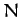point objects interacting via gravity. Let the mass and position vector of theth object be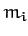and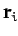, respectively. What is the vector equation of motion of theth object? Write expressions for the total kinetic energy,, and potential energy,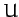, of the system. Demonstrate from the equations of motion that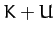is a conserved quantity.

2. Consider a function of many variables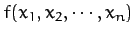. Such a function which satisfies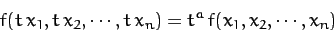for all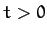, and all values of the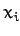, is termed a homogenous function of degree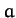. Prove the following theorem regarding homogeneous functions: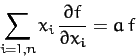3. Consider an isolated system ofpoint objects interacting via attractive central forces. Let the mass and position vector of theth object beand, respectively. Suppose that magnitude of the force exerted on objectby object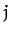is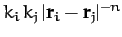. Here, the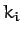measure some constant physical property of the particles (e.g., their electric charges). Write an expression for the total potential energyof the system. Is this a homogenous function? If so, what is its degree? Write the equation of motion of theth particle. Use the mathematical theorem from the previous exercise to demonstrate that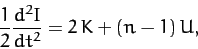where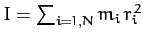, andis the kinetic energy. This result is known as the virial theorem. Demonstrate that there are no bound steady-state equilibria for the system (i.e., states in which the global system parameters do not evolve in time) when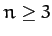.

4. A star can be through of as a spherical system that consists of a very large number of particles interacting via gravity. Show that, for such a system, the virial theorem, introduced in the previous exercise, implies that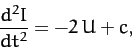where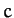is a constant, and the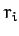are measured from the geometric center. Hence, deduce that the angular frequency of small amplitude radial pulsations of the star (in which the radial displacement is directly proportional to the radial distance from the center) takes the form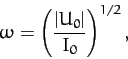where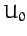and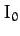are the equilibrium values ofand. Finally, show that if the mass density within the star varies as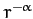, where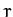is the radial distance from the geometric center, and where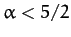, then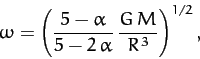where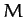and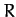are the stellar mass and radius, respectively.

5. Consider a system ofpoint particles. Let theth particle have mass, electric charge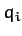, and position vector. Suppose that the charge to mass ratio,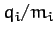, is the same for all particles. The system is placed in a uniform magnetic field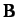. Write the equation of motion of theth particle. You may neglect any magnetic fields generated by the motion of the particles. Demonstrate that the total momentum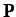of the system precesses aboutat the frequency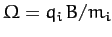. Demonstrate that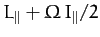is a constant of the motion. Here,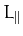is the total angular momentum of the system parallel to the magnetic field, and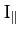is the moment of inertia of the system about an axis parallel towhich passes through the origin.Next: One-Dimensional Motion Up: Newton's Laws of Motion Previous: Non-Isolated Systems
Richard Fitzpatrick 2011-03-31